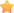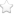×• 8×2=4
• 16×4=4
• 20×5=4
• 10×5=2
• 18×3=6◎本題引用自遊戲學校，網友liuxing8642提供

• 8小時×2=16小時，12小時制中，16時=下午「4時」。
• 16小時×4=64小時=2日又16小時，12小時制中，16時=下午「4時」。
• 20小時×5=100小時=4日又「4小時」。
• 10小時×5=50小時=2日又「2小時」。
• 18小時×3=54小時=2日「6小時」。

（不確定答案怎麼解出來的嗎？快到粉絲團遊戲學校去看看大家的解法吧！）